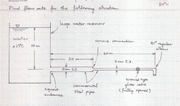# Loss coefficient K in a pipe?

• ipocoyo
The K-factor for each length of straight pipe is f (L/D). Call one K-factor K-3 for the 3-cm. pipe and the other K5 for the 5-cm. pipe.Now, according to the relation##\frac{K_a}{K_b}=(\frac{d_a}{d_b})^4##If you make Ka = K3, then Kb becomes K5. Putting the value of the K-factor for the 5-cm. straight pipe into the relation will give you the equivalent K-factor as if the 5-cm. pipe was actually 3-cm. pipe.

## Homework StatementN/A

## The Attempt at a Solution

Usually we are given a table, but how do we calculate the loss coefficient between 2 different pipes with different diameters? In this case, between the 5 and the 3 cm^2 pipes?

ipocoyo said:

## Homework StatementN/A

## The Attempt at a Solution

Usually we are given a table, but how do we calculate the loss coefficient between 2 different pipes with different diameters? In this case, between the 5 and the 3 cm^2 pipes?
The K-factors for a given diameter of pipe are proportional to the fourth power of that diameter, as described in this article:

http://www.pipeflowcalculations.com/pipe-valve-fitting-flow/flow-in-valves-fittings.php

The usual method is to express the K-factors or equivalents for the system in terms of one common pipe size.

You should be able to look up the equation for the loss coefficient for the transition between two pipes of different diameter. Do you not have a textbook?

Chestermiller said:
You should be able to look up the equation for the loss coefficient for the transition between two pipes of different diameter. Do you not have a textbook?

My textbook just says to refer to appendix for K values. It doesn't say anything between the transition of 2 different pipes with different diameters.

ipocoyo said:
So the answer between the transition of two pipes is (0.03/0/05)^4 ?

The K-factor for each length of straight pipe is f (L/D). Call one K-factor K-3 for the 3-cm. pipe and the other K5 for the 5-cm. pipe.

Now, according to the relation

##\frac{K_a}{K_b}=(\frac{d_a}{d_b})^4##

If you make Ka = K3, then Kb becomes K5. Putting the value of the K-factor for the 5-cm. straight pipe into the relation will give you the equivalent K-factor as if the 5-cm. pipe was actually 3-cm. pipe.

ipocoyo said:
My textbook just says to refer to appendix for K values. It doesn't say anything between the transition of 2 different pipes with different diameters.
What geometries does it give K values for?